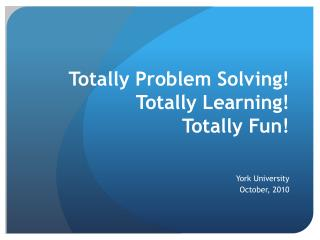DownloadDownload PresentationTotally Problem Solving! Totally Learning! Totally Fun!

# Totally Problem Solving! Totally Learning! Totally Fun!

Télécharger la présentation## Totally Problem Solving! Totally Learning! Totally Fun!

- - - - - - - - - - - - - - - - - - - - - - - - - - - E N D - - - - - - - - - - - - - - - - - - - - - - - - - - -
##### Presentation Transcript

1. Totally Problem Solving! Totally Learning! Totally Fun! York University October, 2010

2. Totally Problem Solving! Totally Learning! Totally Fun! Agenda • Introductions (Favourite Shape) • Elastic bands • Math Path (Problem Posing) • Biggest? • Tangrams • Debrief

3. Totally Problem Solving! Totally Learning! Totally Fun! Elastic Band Geometry • Triangle • Square • Rhombus • A Figure with a Line of Symmetry • Triangular Pyramid (Tetrahedron) • Rectangular Prism

4. Totally Problem Solving! Totally Learning! Totally Fun! Math Path Measurement Kit: • Ruler • String • Centimeter Grid • Pipe Cleaners • Meter Stick • Cups • Piece of Paper • Stop Watch • Weights

5. Totally Problem Solving! Totally Learning! Totally Fun! Which is the biggest? Justify your answer. • Oak Leaves • Cosmos • Pine Cones

6. Totally Problem Solving! Totally Learning! Totally Fun! Constructing Your Own Set of Tangrams by Tom Scavo Materials • a rectangular piece of paper suitable for folding • a pair of scissors • a ruler (optional) A complete set of tangrams consists of seven pieces: • a small square • two small congruent triangles • two large congruent triangles • a medium-size triangle • a parallelogram You can make your own set of tangrams from a single piece of paper.

7. Totally Problem Solving! Totally Learning! Totally Fun! http://www.tangrams.ca/

8. Totally Problem Solving! Totally Learning! Totally Fun! • What is problem solving? • How are the processes of mathematics embedded in problem solving? • What is a problem solving approach to teaching? (Generating Curiosity in Mathematics Learning) • Why use a problem solving approach? • Sources: OAME Gazette, NCTM Teaching Children Mathematics